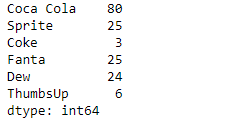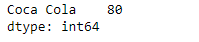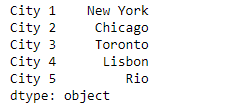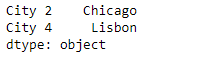Open in App
Not now

# Python | Pandas Series.filter()

• Last Updated : 13 Feb, 2019

Pandas series is a One-dimensional ndarray with axis labels. The labels need not be unique but must be a hashable type. The object supports both integer- and label-based indexing and provides a host of methods for performing operations involving the index.

Pandas` Series.filter()` function returns subset rows or columns of dataframe according to labels in the specified index. Please note that this routine does not filter a dataframe on its contents. The filter is applied to the labels of the index.

Syntax: Series.filter(items=None, like=None, regex=None, axis=None)

Parameter :
items : List of axis to restrict to (must not all be present).
like : Keep axis where “arg in col == True”.
regex : Keep axis with re.search(regex, col) == True.
axis : The axis to filter on. By default this is the info axis, ‘index’ for Series, ‘columns’ for DataFrame.

Returns : same type as input object

Example #1: Use `Series.filter()` function to filter out some values in the given series object using a regular expressions.

 `# importing pandas as pd``import` `pandas as pd`` ` `# Creating the Series``sr ``=` `pd.Series([``80``, ``25``, ``3``, ``25``, ``24``, ``6``])`` ` `# Create the Index``index_ ``=` `[``'Coca Cola'``, ``'Sprite'``, ``'Coke'``, ``'Fanta'``, ``'Dew'``, ``'ThumbsUp'``]`` ` `# set the index``sr.index ``=` `index_`` ` `# Print the series``print``(sr)`

Output :Now we will use `Series.filter()` function to filter those values from the given series object whose index label name has a space in its name.

 `# filter values``result ``=` `sr.``filter``(regex ``=` `'. .'``)`` ` `# Print the result``print``(result)`

Output :As we can see in the output, the `Series.filter()` function has successfully returned the desired values from the given series object.

Example #2 : Use `Series.filter()` function to filter out some values in the given series object using a list of index labels.

 `# importing pandas as pd``import` `pandas as pd`` ` `# Creating the Series``sr ``=` `pd.Series([``'New York'``, ``'Chicago'``, ``'Toronto'``, ``'Lisbon'``, ``'Rio'``])`` ` `# Create the Index``index_ ``=` `[``'City 1'``, ``'City 2'``, ``'City 3'``, ``'City 4'``, ``'City 5'``] `` ` `# set the index``sr.index ``=` `index_`` ` `# Print the series``print``(sr)`

Output :Now we will use `Series.filter()` function to filter the values corresponding to the passed index labels in the given series object.

 `# filter values``result ``=` `sr.``filter``(items ``=` `[``'City 2'``, ``'City 4'``])`` ` `# Print the result``print``(result)`

Output :As we can see in the output, the `Series.filter()` function has successfully returned the desired values from the given series object.

My Personal Notes arrow_drop_up